# NCERT Solutions for Class 7 Maths Chapter 3 Data Handling InText Questions

These NCERT Solutions for Class 7 Maths Chapter 3 Data Handling InText Questions and Answers are prepared by our highly skilled subject experts.

## NCERT Solutions for Class 7 Maths Chapter 3 Data Handling InText Questions

NCERT In-text Question Page No. 59
Question 1.
Weigh (in kg) at least 20 children (girls and boys) of your class. Organise the date, and answer the following questions using this data.
(i) Who is the heaviest of all?
(ii) What is the most common weight?
(iii) What is the difference between your weight and that of your best friend?
We assume that weights (in kg) of 20 children of the class are as follows:
27, 30, 28, 24, 25, 26, 21, 20, 18, 15,
28, 16, 27, 23, 24, 26, 22, 31, 18, 26
Organising the data in ascending order, we have.
15, 16, 18, 18, 20, 21, 22, 23, 24, 24,
25, 26, 26, 27, 27, 28,28, 30,31

(i) The child having weight 31 kg is the heaviest of all.
(ii) The most common weight is 26 Kg.
(iii) If my weight is 27, then required difference = 27 kg – 25 Kg = 2 Kg.NCERT In-text Question Page No. 61
Question 1.
How would you find the average of your study hours for the whole week?
In a week there are 7 days. The number of study hours everyday are different. Let the study hours for different days of the week are as follows:
Monday : 6 hours
Tuesday : 7 hours
Wednesday : 6 hours
Thursday : 8 hours
Friday : 8 hours
Saturday : 7 hours
Sunday : 7 hours
Total number of study hours.
= 6 + 7 + 6 + 8 + 8 + 7 + 7 = 40 hours
Number of total days = 7
∴ Arithmetic mean = $$\frac{\text { Total study hours }}{\text { Total Number of days }}$$
Thus, average study hours = 7 hours per day.

NCERT In-text Question Page No. 61
Question 1.
Find the mean of your sleeping hours daring one week?
Let the sleeping hours be as follows:
Monday : 8 hours
Tuesday : 9 hours
Wednesday : 11 hours
Thursday : 8 hours
Friday : 10 hours
Saturday : 9 hours
Sunday : 8 hours
Sum of sleeping hours
= 8 + 9+11 + 8+10 + 9 + 8 = 63
Number of days = 7
∴ Arithmetic mean = $$\frac{\text { Sum of sleeping hours }}{\text { Number of days }}$$
= $$\frac{63}{7}$$ hours = 9hoursQuestion 2.
Find at least 5 numbers between $$\frac{1}{2}$$ and $$\frac{1}{3}$$.
The arithmetic mean of two given num¬bers is average between them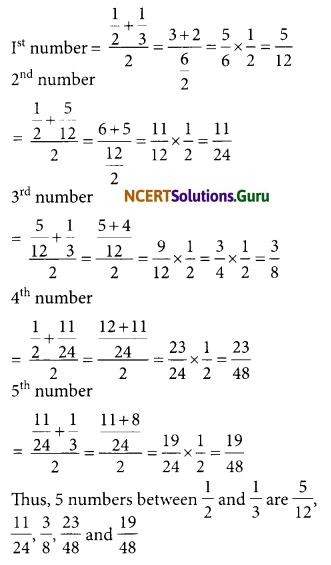NCERT In-text Question Page No. 65
Question 1.
Find the mode of?
(i) 2, 6, 5, 3, 0, 3,4, 3, 2, 4, 5, 2, 4
(ii) 2, 14,16,12,14,14, 16, 14,10, 14,18,14
(i) Given set of data is :
2, 6, 5, 3, 0, 3, 4, 3, 2, 4, 5, 2, 4
Writing the numbers with same value together, we have:
0, 2, 2, 2, 3, 3, 3, 4, 4, 4, 5, 5, 6
∵ 2,3, and 4 occur in the given data highest times (3 times).
∴ Modes of the set of data are 2, 3 and 4.

(ii) Given set of data is :
2, 14, 16, 12, 14, 14, 16, 14, 10, 14, 18, 14
Writing the numbers with same value together, we have:
2, 10, 12, 14, 14, 14, 14, 14, 14, 16, 16, 18
∵ 14 occurs highest times (i.e. six times).
∴ Required mode is 14.NCERT In-text Question Page No. 65-66
Question 1.
Find the mode of the following data.
12, 14, 12, 16, 15, 13, 14, 18, 19, 12, 14, 15, 16, 15, 16, 16, 15, 17, 13, 16, 16, 15, 15, 13, 15, 17, 15, 14, 15, 13, 15, 14
Here the given set of data has large number of observations so, we put them in a table
Number Tally Marks Frequency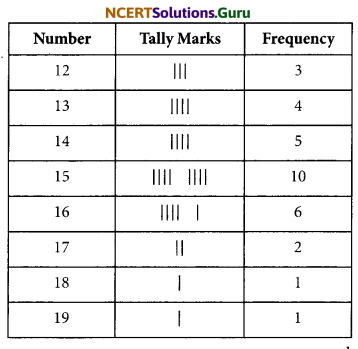The highest frequency is 10, corresponding value is 15.
The required mode is 15.

Question 2.
Heights (in cm) of 25 children are given below:
168, 165, 163, 160, 163, 161, 162, 164, 163, 162, 164, 163, 160, 164, 163, 160, 165, 163, 162
What is the mode of their heights? What do we understand by mode here?
Putting the data ina tabular form, we haveSince, 163 is occuring the maximum number of times.
∴ Mode is 163 cm.
By the mode, we mean that most of stu¬dents have height 163 cm.

Note :
Mode is modal value of a group which occurs most frequently in the data whereas mean gives us the average of all observations of the data.NCERT In-text Question Page No. 66
Question 1.
Discuss with your friends and give:
(a) Two situations where mean would be an appropriate representative value to use, and
(b) Two situations where mode would be an appropriate representative value to use.
(a) (i) For measures of heights of all students of a class, and
(ii) For weights of wheat stored in similar containers, the mean would give us a better picture of the data.

(b) (i) If we are collecting the shoe size suitable for a particular age, and
(ii) A shopkeeper selling shirts needs to know suitable size to enrich the stock, then mode is more useful.

NCERT In-text Question Page No. 67
Question 1.
Your friend found the median and the mode of a given data. Describe and correct your friend’s error if any:
35, 32, 35, 42, 38, 32, 34
Median = 42, Mode = 32
Set of data is: 35, 32, 35, 42, 38, 32, 34
Putting the given data in ascending order, we have:
32, 32, 34, 35, 35, 38, 42

(i)∵ The middle value of the data is 35.
∴ Median = 35

(ii)∵ The observations occuring maximum number of times are 32 and 35.
∴ The correct modes are 32 and 35.NCERT In-text Question Page No. 71 & 72
Question 1.
The bar graph given below shows the result of a survey to test water resistant watches made by different companies.
Each of these companies claimed that their watches were water resistant. After a test the above results were revealed.
(a) Can you work out a fraction of the number of watches that leaked to the number tested for each company?
(b) Could you tell on this basis which company has better watches?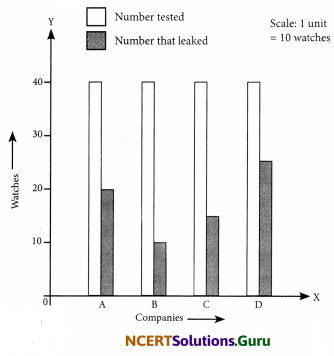(a) From the graph, we have :
Total number of watches tested for each company = 40
Fraction of ‘the numbers of watches that leaked’ to ‘the number of watches tested’ 20 1
For company A = $$\frac{20}{40}=\frac{1}{2}$$
For company B = $$\frac{10}{40}=\frac{1}{4}$$
For company C = $$\frac{15}{40}=\frac{3}{8}$$
For company D = $$\frac{25}{40}=\frac{5}{8}$$
(b) Obviously company B has better watches
∵ $$\frac { 1 }{ 4 }$$ < $$\frac { 3 }{ 8 }$$ > $$\frac { 1 }{ 2 }$$ < $$\frac { 5 }{ 8 }$$

Question 2.
Sale of English and Hindi books in the years 1995, 1996, 1997 and 1998 are given below: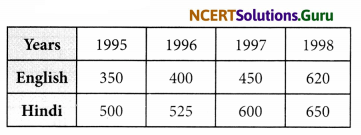Draw a double bar graph and answer the following questions:
(a) In which year was the difference in the sale of the two language books least?
(b) Can you say that the demand for English books rose faster? Justify.
(a) The difference in the sale of the two language books:
In 1995 is 500 – 350 = 150
In 1996 is 525 – 400 = 125
In 1997 is 600 – 450 = 150
In 1998 is 650 – 620 = 30
The difference in the scale of the two language books is least in the year 1998.

(b) Yes we can say that the demand of English books rose faster, during 1995 to 1998
(i) Sale of Hindi books rose from 500 to 650 i.e. it rose by 650 – 500 = 150
(ii) Sale of English books rose from 350 to 620 i.e. it rose by 620 – 350 = 270
270 is much greater than 150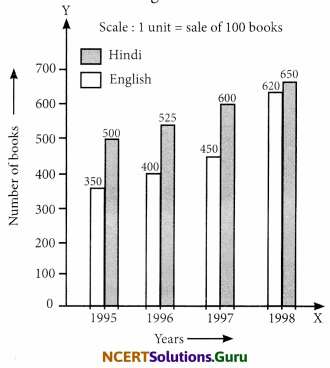NCERT In-text Question Page No. 74
Question 1.
Think of some situations, atleast 3 examples of each, that are certain to happen, some that are impossible and some that may or may not happen i.e., situations that have some chance of happening.
(i) Situations that are certain to happen
(a) The sun rises in the east.
(b) Getting a number from 1 to 6 by throwing a dice.
(c) When a coin is tossed, then either a head or tail comes.

(ii) Situations that are impossible:
(a) Getting the number 8 by throwing a dice.
(b) To draw a five rupee coin from a bag containing one rupee coins.
(c) Drawing a red ball from a bag containing yellow and blue balls only.

(iii) Situations that have some chance of happening
(a) An ant rising to 5m height.
(b) To throw a dice and get an odd number.
(c) To toss a coin and get tail.

Note:
(i) A coin has two faces. The face with Ashoka Chakra is called ‘Head’ and other is called ‘Tail’.
(ii) A cube with six faces is called a dice. Its each face has making of dots numbering from 1 to 6.

NCERT In-text Question Page No. 75
Question 1.
Toss a coin 100 times and record the data. Find the number of times heads and tails occur in it.
This is a group activity. Please do it yourself.

Question 2.
Aftaab threw a die 250 times and got the following table. Draw a bar graph for this data.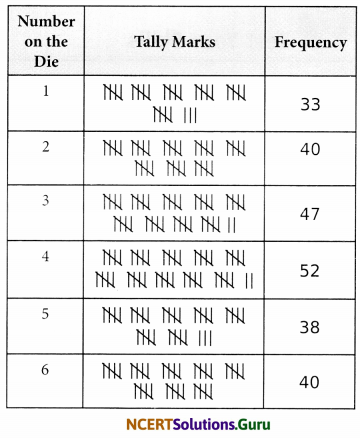NCERT In-text Question Page No. 76
Question 1.
Construct or think of five situations where outcomes do not have equal chances.